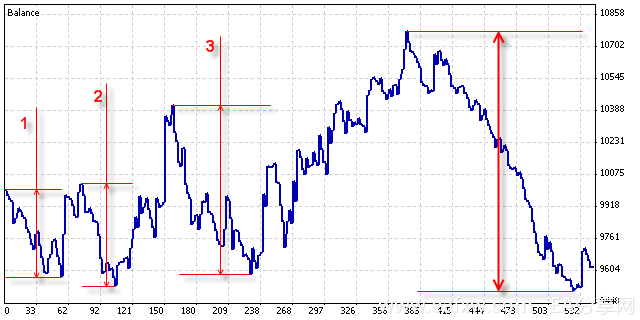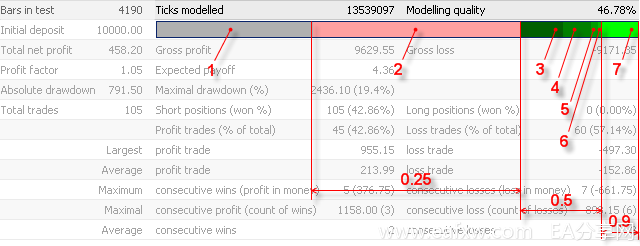'Bars in test' 以模型为基础,显示历史的深度。
'Ticks modelled'显示模型次序的大小。 每一个记录的次序代表柱的当前或另一时刻状态 (OHLCV)。 不同柱的状态取决于时间范围，模型方法，和从较小时间段内的柱的历史数据。
'Modelling quality' 按照以下的公式进行计算：
ModellingQuality = ((0.25*(StartGen-StartBar) +
0.5 *(StartGenM1-StartGen) +
0.9 *(HistoryTotal-StartGenM1)) / (HistoryTotal-StartBar))*100%;

HistoryTotal – 在历史中的总数额；
StartBar – 开始测试柱的数字。模型开始于最小的第101个柱或者测试水平初始日期相关的柱;
StartGen – 在最近的时间范围内开始测试柱的数字；
StartGenM1 – 在原有分钟内开始测试柱的数字；

Gross profit, 所有赢利交易总数的净赢利值;
Gross loss, 所有亏损交易总数的净亏损值；
Total net profit, 净赢利值和净亏损值之间的差别:
TotalNetProfit = GrossProfit – GrossLoss
Profit factor, 赢利原因显示在多少时间内净赢利值超过净亏损值:
ProfitFactor = GrossProfit / GrossLoss
Expected payoff,预期值可以使用以下公式进行计算:

GrossProfit – 净赢利交易总数;
GrossLoss – 净亏损交易总数.

AbsoluteDrawDown = InitialDeposit – MinimalBalance

MaximalDrawDown = Max of (Maximal Peak – next Minimal Peak)MaxDrawDown % = MaxDrawDown / its MaxPeak * 100%

Short positions (won %) – 卖空仓位总数额和其中赢利百分比（卖空仓位/卖空仓位总数*100%）；
Long positions (won %) – 看涨仓位总数额和其中赢利百分比（看涨仓位/看涨仓位总数*100%）；
Profit trades (% of total) – 赢利交易总数和交易总数的百分比（赢利交易/交易总数*100%）；
Loss trades (% of total) – 亏损交易总数和交易总数的百分比（亏损交易/交易总数*100%）；
Average profit trade – 赢利交易中赢利的平均数 (净赢利值 / 赢利交易)；
Average loss trade – 亏损交易中亏损的平均数(净亏损值 / 亏损交易)；
Maximum consecutive wins (profit in money) – 在这一系列赢利总数和交易的赢利系列中最大连续盈利；
Maximum consecutive losses (loss in money) – 在这一系列亏损总数和交易的亏损系列中最大连续损失；
Maximal consecutive profit (count of wins) – 在交易总数中最大连续交易的赢利；
Maximal consecutive loss (count of losses) – 在交易总数中最大连续交易的赢利；
Average consecutive wins – 赢利系列中连续盈利的平均数；
Average consecutive losses – 亏损系列中连续损失的平均数.Bars in test = 4190;
StartBar = 2371;
StartGen (H4) = 3042 (图中标注为3 );
Start H1 = 3355 (图中标注为4);
Start M30 = 3841 (图中标注为5);
Start M15 = 3891 (图中标注为6);
Start M5 = 0 (图中没有标注);
Start M1 = 3917.

((0.25*(3042-2371) + 0.5*(3917-3042) + 0.9*(4190-3917)) / (4190-2371))*100% =
((0.25*671 + 0.5*875 + 0.9*273) / 1819)*100%                                                 = 46.78%